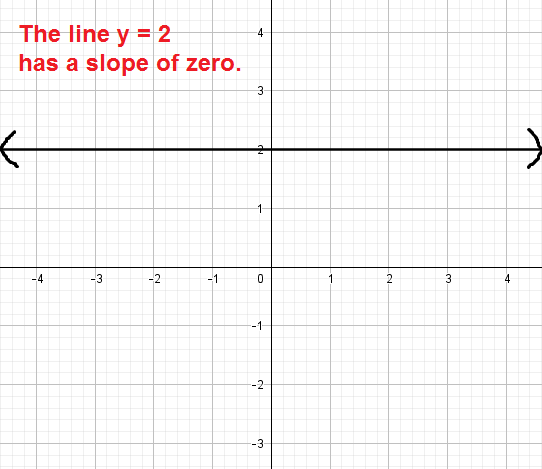# Zero slope

When you understand that the slope of a line is a way of describing the “steepness” of a line, it is easy to see why a line may have positive or negative slope. But why might a line have zero slope, and what kinds of lines will always have a slope of zero? We will explore these ideas in the following lesson.

## What kinds of lines have zero slope?

Any line of the form $$y = c$$, where $$c$$ is some real number, will have a slope of zero. The lines are horizontal (flat) and so have no “steepness” to measure.To understand the discussion below, you should be familiar with finding the slope using the slope formula.

## Why do horizontal lines have slopes of zero?

Let’s use the line graphed above, $$y = 2$$, to understand why a line like this will always have zero slope. Pick two points on the line from the graph, say $$(1, 2)$$ and $$(2,2)$$. We can let $$(x_1, y_1) = (1,2)$$ and $$(x_2, y_2) = (2,2)$$ and apply the slope formula:

\begin{align}\text{slope} &= \dfrac{y_2 – y_1}{x_2 – x_1}\\ &= \dfrac{2 – 2}{2 – 1} \\&= \dfrac{0}{1} \\&= 0\end{align}

Every point on the line $$y = 2$$ has a y-coordinate of 2. So no matter which two points we pick, we will end up with zero in the numerator when applying the slope formula.

For other vertical lines, $$y = c$$, the y-coordinates will always be $$c$$. So, the same idea applies and the slope will always end up being zero.

IMPORTANT: the fraction $$\dfrac{0}{c}$$ is 0 for any nonzero number $$c$$. But, the fraction $$\dfrac{c}{0}$$ is always undefined.

• Undefined – the line is vertical (of the form $$x = c$$). (See lesson: undefined slope)